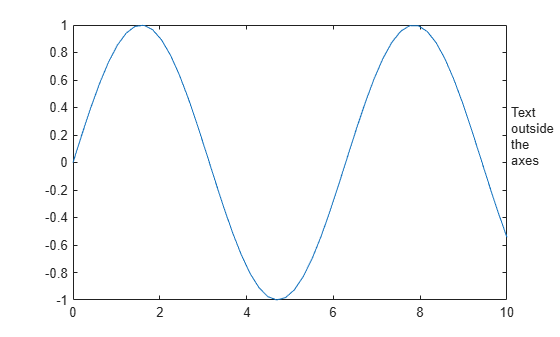# Add Text to Chart

This example shows how to add text to a chart, control the text position and size, and create multiline text.

### Text Position

Add text next to a particular data point using the text function. In this case, add text to the point $\left(\pi ,\mathrm{sin}\left(\pi \right)\right)$. The first two input arguments to the text function specify the position. The third argument specifies the text.

By default, text supports a subset of TeX markup. Use the TeX markup \pi for the Greek letter $\pi$. Display an arrow pointing to the left by including the TeX markup \leftarrow. For a full list of markup, see Greek Letters and Special Characters in Chart Text.

x = linspace(0,10,50); y = sin(x); plot(x,y) txt = '\leftarrow sin(\pi) = 0'; text(pi,sin(pi),txt)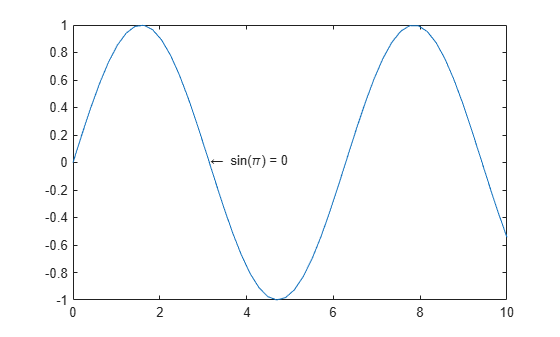### Text Alignment

By default, the specified data point is to the left of the text. Align the data point to the right of the text by specifying the HorizontalAlignment property as 'right'. Use an arrow pointing to the right instead of to the left.

x = linspace(0,10,50); y = sin(x); plot(x,y) txt = 'sin(\pi) = 0 \rightarrow'; text(pi,sin(pi),txt,'HorizontalAlignment','right')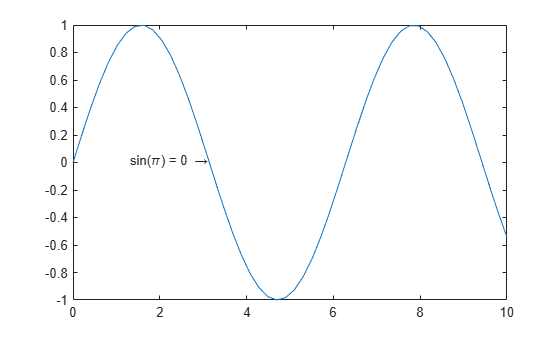### Font Size

Specify the font size for text by setting the FontSize property as a name-value pair argument to the text function. You can use a similar approach to change the font size when using the title, xlabel, ylabel, or legend functions.

x = linspace(0,10,50); y = sin(x); plot(x,y) txt = '\leftarrow sin(\pi) = 0'; text(pi,sin(pi),txt,'FontSize',14)Alternatively, starting in R2022a, you can change the font size of the axes text by using the fontsize function.

### Setting Text Properties

The text function creates a Text object. Text objects have properties that you can use to customize the appearance of the text, such as the HorizontalAlignment or FontSize.

You can set properties in two ways:

• Use name-value pairs in the text command, such as 'FontSize',14.

• Use the Text object. You can return the Text object as an output argument from the text function and assign it to a variable, such as t. Then, use dot notation to set properties, such as t.FontSize = 14.

For this example, change the font size using dot notation instead of a name-value pair.

x = linspace(0,10,50); y = sin(x); plot(x,y) txt = '\leftarrow sin(\pi) = 0'; t = text(pi,sin(pi),txt)
t = Text (\leftarrow sin(\pi) = 0) with properties: String: '\leftarrow sin(\pi) = 0' FontSize: 10 FontWeight: 'normal' FontName: 'Helvetica' Color: [0 0 0] HorizontalAlignment: 'left' Position: [3.1416 1.2246e-16 0] Units: 'data' Show all properties 
t.FontSize = 14;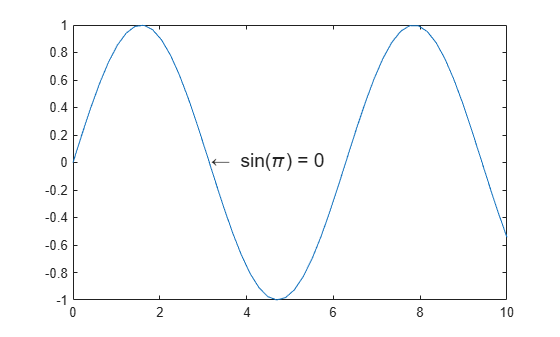### Multiline Text

Display text across multiple lines using a cell array of character vectors. Each element of the cell array is one line of text. For this example, display a title with two lines. You can use a similar approach to display multiline text with the title, xlabel, ylabel, or legend functions.

x = linspace(0,10,50); y = sin(x); plot(x,y) txt = {'Plotted Data:','y = sin(x)'}; text(4,0.5,txt)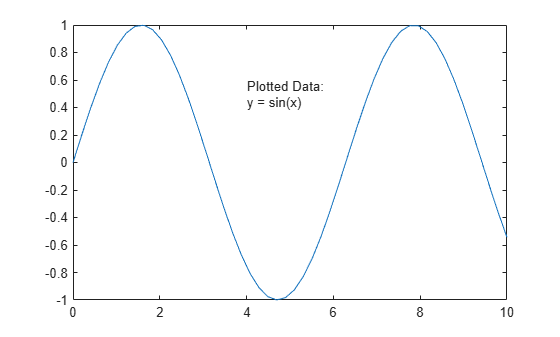### Text with Variable Value

Include a variable value in text by using the num2str function to convert the number to text. For this example, calculate the average y value and include the value in the title. You can use a similar approach to include variable values with the title, xlabel, ylabel, or legend functions.

x = linspace(0,10,50); y = sin(x); plot(x,y) avg = mean(y); txt = ['Average height: ' num2str(avg) ' units']; text(4,0.5,txt)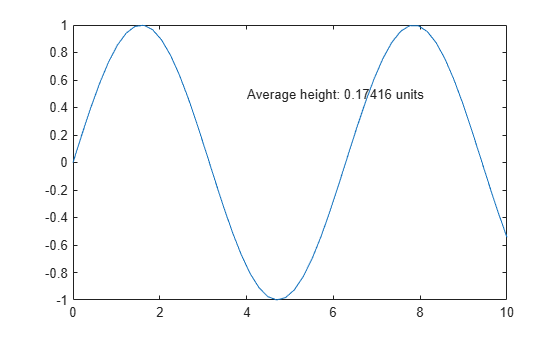### Text Outside Axes

Add text anywhere within the figure using the annotation function instead of the text function. The first input argument specifies the type of annotation. The second input argument specifies the position of the annotation in units normalized to the figure. Remove the text box border by setting the EdgeColor property to 'none'. For more information on text box annotations, see the annotation function.

x = linspace(0,10,50); y = sin(x); plot(x,y) annotation('textbox',[.9 .5 .1 .2], ... 'String','Text outside the axes','EdgeColor','none')